Courses

# NCERT Exemplar (Part - 1) - Haloalkanes and Haloarenes Notes | EduRev

## Class 12 : NCERT Exemplar (Part - 1) - Haloalkanes and Haloarenes Notes | EduRev

The document NCERT Exemplar (Part - 1) - Haloalkanes and Haloarenes Notes | EduRev is a part of the Class 12 Course Chemistry Class 12.
All you need of Class 12 at this link: Class 12

MULTIPLE CHOICE QUESTIONS (TYPE - I)

Q.1. The  order of reactivity of following alcohols with halogen acids is ________.
(A) CH3CH2—CH2—OH
(B)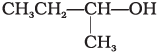(C)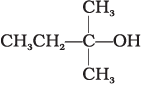(i) (A) > (B) > (C)
(ii) (C) > (B) > (A)
(iii) (B) > (A) > (C)
(iv) (A) > (C) > (B)
Ans. (ii)
Solution.
The reactivity of alcohols depends on the type of alcohol (ie.primary / secondary / tertiary). It is due to the presence of nature of carbocation present in its structure
Higher the stability of carbocation, higher will be the possibilities of attack of X ion to the carbocation. The order of reactivity of alcohols with a given haloacid is acid is 3º >2º >1º.
So, the correct option is (ii).

Q.2. Which of the following alcohols will yield the corresponding alkyl chloride on reaction with concentrated HCl at room temperature?
(i) CH3CH2—CH2—OH
(ii)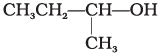(iii)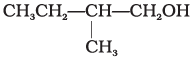(iv)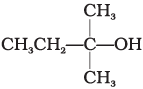Ans. (iv)
Solution.

When alcohols are treated with conc. HCl at room temperature than alkyl chloride is formed. This reaction follows SN1 mechanism SN1 mechanism completes in two steps. In first step, a carbocation is formed and is attacked by nucleophile in second step.
The attack of nucleophile to the carbocation is possible only if the carbocation is stable. Compound present in option (iv) will give tertiary carbocation in step 1. Tertiary carbocation is the most stable so it is further attacked by Cl nucleophile as follows.
Step I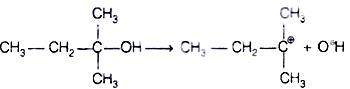Step II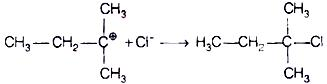Q.3. Identify the compound Y in the following reaction.(i)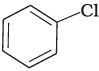(ii)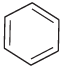(iii)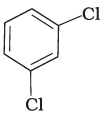(iv)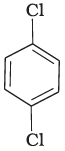Ans. (i)
Solution.

When a primary aromatic amine is dissolved or suspended in cold aqueous mineral acid and treated with sodium nitrite, a diazonium salt is formed. When this freshly prepared diazonium salt is mixed with cuprous chloride, then diazonium group is replaced by Cl atom. Then chlorobenzene is formed which is y in this reaction.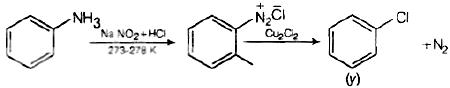Q.4. Toluene reacts with a halogen in the presence of iron (III) chloride giving ortho and para halo compounds. The reaction is
(i) Electrophilic elimination reaction
(ii) Electrophilic substitution reaction
(iv) Nucleophilic substitution reaction
Ans. (ii)
Solution.

Toluene reacts with a halogen in the presence of iron (III) chloride giving ortho and para halo compounds. The reaction is electrophilic substitution reaction.
It has the following mechanism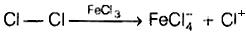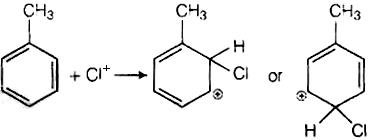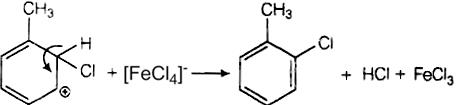In this mechanism, electrophile Cl+ attacks to electron rich benzene ring and replaces hydrogen. So, the reaction is electrophilic substitution reaction.

Q.5. Which of the following is halogen exchange reaction?
(i) RX + NaI → RI + NaX
(ii)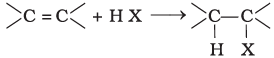(iii)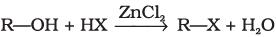(iv)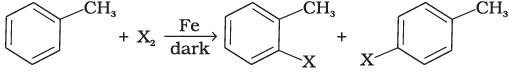Ans. (i)
Solution.
Halogen exchange reactions are those reactions in which one halide replaces another. In option (i) halogen (−X) is replaced by iodine. This reaction is named as Finkelstein reaction.
In option (ii), there is the addition of hydrogen halide on alkene.
In option (iii), halogen replaces alcoholic group.
While in option (iv) halogen replaces the hydrogen of benzene line.

Q.6. Which reagent will you use for the following reaction?
CH3CH2CH2CH3 → CH3CH2CH2CH2Cl + CH3CH2CHClCH3
(i) Cl2/UV light
(ii) NaCl + H2SO4
(iii) Cl2 gas in dark
(iv) Cl2 gas in the presence of iron in dark

Ans. (i)
Solution.

The given reaction is a substitution reaction. It involves the replacement of 1° or 2° hydrogen of alkanes by chlorine. It occurs in presence of ultraviolet light or at high temperature.
The chlorination does not occur at room temperature in absence of light. In this reaction, light is absorbed by the chlorine molecule and activated chlorine initiates the reaction as follows
Step 1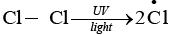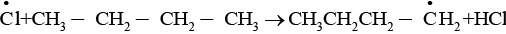Step 2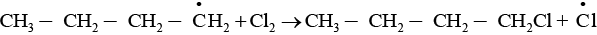Step 3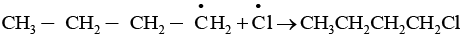So, option (i) is the correct

Q.7. Arrange the following compounds in the increasing order of their densities.
(a)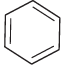(b)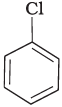(c)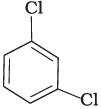(d)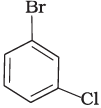(i) (a) < (b) < (c) <  (d)
(ii) (a) < (c) < (d) <  (b)
(iii) (d) < (c) < (b) <  (a)
(iv) (b) < (d) < (c) <  (a)

Ans. (i)
Solution.

Density is directly related to molecular mass. Higher the molecular mass, higher will be the density of the compound. Among the four given compounds, the order of molecular mass is benzene < chlorobenzene < dichlorobenzene < bromochlorobenzene
Therefore, the increasing order of their densities is same as above.
Hence, option (i) is correct.

Q.8. Arrange the following compounds in increasing order of their boiling points.
(a)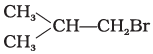(b) CH3CH2CH2CH2Br
(c)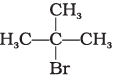(i) (b) < (a) < (c)
(ii) (a) < (b) < (c)
(iii) (c) < (b) < (d)
(iv) (c) < (b) < (a)

Ans. (iii)
Solution.

Boiling point of a compound depends upon the surface area. Higher the surface area higher will be the boiling point of a compound. Surface area decreases with increase in branching in a molecule, so its boiling point will also decrease.
Hence, option (iii) is correct.

Q.9. In which of the following molecules carbon atom marked with asterisk (*) is asymmetric?
(a)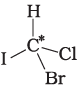(b)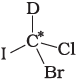(c)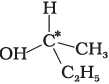(d)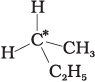(i) (a), (b), (c), (d)
(ii) (a), (b), (c)
(iii) (b), (c), (d)
(iv) (a), (c), (d)
Ans. (ii)
Solution.

Asymmetric/chiral carbon atom is that in which all of its four valencies with four different groups or atoms. In compound (iv), carbon satisfies two of its valencies with two hydrogen atoms i,e. similar atom.
So, it is not an asymmetric carbon atom while rest of the three molecules have asymmetric carbon as each carbon has satisfied all four valencies with four different groups or atoms.
So, the correct option is (ii).

Q.10. Which of the following structures is enantiomeric with the molecule (A) given below :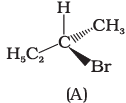(i)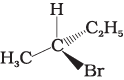(ii)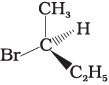(iii)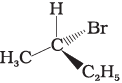(iv)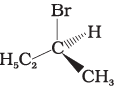Ans. (i)
Solution.

The stereoisomers related to each other as non-superimposable mirror images are called enantiomers. Enantiomers possess identical physical properties. They only differ with respect to the rotation of plane polarised light. If one of the enantiomer is dextro rotatory, the other will be laevo rotatory. Here, the enantiomer of molecule (A) is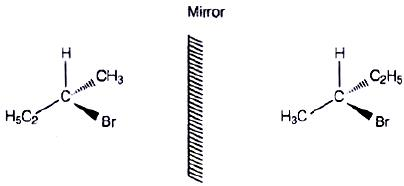Hence, option (i) is correct.

Q.11. Which of the following is an example of vic-dihalide?
(i) Dichloromethane
(ii) 1, 2-dichloroethane
(iii) Ethylidene chloride
(iv) Allyl chloride
Ans. (ii)
Solution.

vic-dihalides are those halides in which two halogen atoms are present on the two adjacent carbon atoms.
Structures of the given compounds are as follows: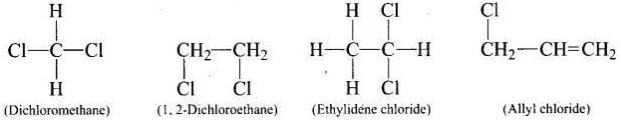In 1, 2-dichloroethane, the two chlorine atoms are attached to two adjacent carbon atoms.
Hence, option (ii) is correct.

Q.12. The position of –Br in the compound in CH3CH = CHC(Br)(CH3)2 can be classified as ____________.
(i) Allyl
(ii) Aryl
(iii) Vinyl
(iv) Secondary

Ans. (i)
Solution.

Allyl halides are those compounds in which the halogen atom is bonded to sp3 hybridised carbon atom next to carbon carbon-double bond.
e.g., CH2 = CH —CH2X and CH3CH = CHC(Br) (CH3)2.
Aryl halides are the compounds in which the halogen atom is bonded to the sp2 hybridised carbon atom of an aromatic ring.
e.g.,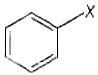Vinyl halides are the compounds in which the halogen atom is bonded to the sp2 hybridised carbon atom of a carbon double bond.
e.g.,
CH2 = CH—X
Secondary alkyl halides are the compounds in which the halogen atom is bonded to the sp3 hybridised carbon atom which is further bonded to two alkyl groups and one hydrogen atom
e.g.,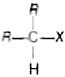Q.13. Chlorobenzene is formed by reaction of chlorine with benzene in the presence of AlCl3. Which of the following species attacks the benzene ring in this reaction?
(i) Cl
(ii) Cl+
(iii) AlCl3
(iv) [AlCl4]
Ans. (ii)
Solution.

In this reaction, AlCl3 is a catalyst which activates the chlorine molecule to show heterolytic cleavage. AlCl3 is electron deficient molecule and forms AlCl4 and Cl+ when reacts with Cl2. This Cl+ electrophile attacks on electron rich benzene ring.
AlCl3 + Cl2 → [AlCl4] + Cl+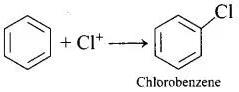Q.14. Ethylidene chloride is a/an __________.
(i) Vic-dihalide
(ii) Gem-dihalide
(iii) Allylic halide
(iv) Vinylic halide
Ans. (ii)
Solution.

In vic-dihalides, halogen atoms are present on the adjacent carbon atoms.
In gem-dihalides, halogen atoms are present on the same carbon atom. They are known as alkylidene halides
In allylic halides, halogen atom is bonded to sp3 hybridised carbon atom next to carbon-carbon double bond.
In vinylic halides, halogen atom is bonded to sp2 hybridised carbon atom of a carbon-carbon double bond.
In ethylidene chloride (H3C—CHCl2) both halogen atoms are present on same carbon atom so it is gem-dihalide.

Q.15. What is ‘A’ in the following reaction?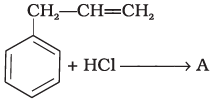(i)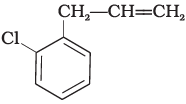(ii)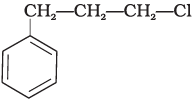(iii)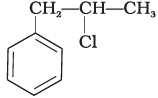(iv)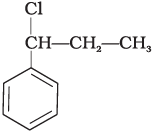Ans. (iii)
Solution.

In this reaction, addition of HCl takes place on doubly bonded carbons in accordance with Markownikoff's rule i.e., addition of negative addendum will take place on that carbon which has lesser number of hydrogen.
Thus,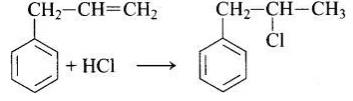Hence, option (iii) is correct.

Q.16. A primary alkyl halide would prefer to undergo ___________.
(i) SN1 reaction
(ii) SN2 reaction
(iii) α–Elimination
(iv) Racemisation
Ans. (ii)
Solution.

A primary alkyl halide would prefer to undergo SN2 reaction.
(a) SN1 reactions occur only if the intermediate carbonation is stable i.e., 3° carbocation.
(b) SN2 reactions occur if there is less steric hinderance on the α-carbon of alkyl halide. In case of primary alkyl halides carbocation is highly unstable and steric hinderance is very less. So, primary alkyl halide would prefer to undergo SN2 reaction.
(c) In α-elimination, proton and the leaving group are present on same atom.
(d) Racemisation is the process of conversion of enantiomer into a racemic mixture.

Q.17. Which of the following  alkyl halides will undergo SN1 reaction most readily?
(i) (CH3)3C—F
(ii) (CH3)3C—Cl
(iii) (CH3)3C—Br
(iv) (CH3)3C—I

Ans. (iv)
Solution.

All the given compounds are tertiary alkyl halides but the bond formed between carbon and iodine (C—I) bond is the weakest bond due to large difference in the size of carbon and iodine. So, (CH3)3C—I gives SN1 reaction most readily. In other words, iodine is a better leaving group.

Q.18. Which is the correct IUPAC name for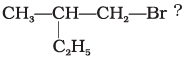(i) 1-Bromo-2-ethylpropane
(ii) 1-Bromo-2-ethyl-2-methylethane
(iii) 1-Bromo-2-methylbutane
(iv) 2-Methyl-1-bromobutane
Ans. (iii)
Solution.

The correct IUPAC name of the given compound is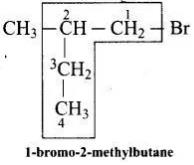Q.19. What should be the correct IUPAC name for diethyl bromomethane?
(i) 1-Bromo-1,1-diethylmethane
(ii) 3-Bromopentane
(iii) 1-Bromo-1-ethylpropane
(iv) 1-Bromopentane
Ans. (ii)
Solution.

Structure of the diethyl bromomethane is given below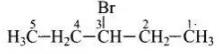So, the IUPA name is 3-bromopentane.

Q.20. The reaction of toluene with chlorine in the presence of iron and in the absence of light yields __________.
(i)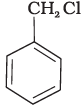(ii)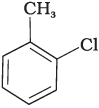(iii)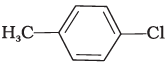(iv) Mixture of (ii) and (iii)
Ans. (iv)
Solution.

Both ortho – and para – compounds are formed in different yields as depicted below-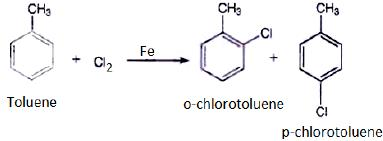Q.21. Chloromethane on treatment with excess of ammonia yields mainly
(i) N, N-Dimethylmethanamine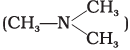(ii) N–methylmethanamine (CH3—NH—CH3)
(iii) Methanamine (CH3NH2)
(iv) Mixture containing all these in equal proportion
Ans. (iii)
Solution.

Chloromethane on treatment with excess of ammonia yields mainly methamine.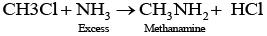However, if the two reactants are present in the same amount, then the mixture of primary, secondary and tertiary amine is obtained.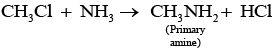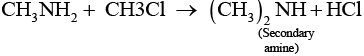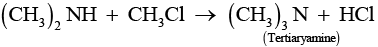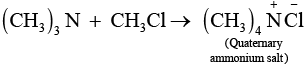Q.22. Molecules whose mirror image is non superimposable over them are known as chiral. Which of the following molecules is chiral in nature?
(i) 2-Bromobutane
(ii) 1-Bromobutane
(iii) 2-Bromopropane
(iv) 2-Bromopropan-2-ol
Ans. (i)
Solution.

Molecules whose mirror image is non-super imposable over them are known as chiral. It occurs on that molecule which contains asymmetric carbon or chiral (i.e., a carbon atom attached with four different groups). In the present case a molecule of 2- Bromobutane [CH3 CH2 C*H (Br) CH3] is a chiral molecule because,
(i) It contains a chiral carbon atom marked as (C*) .
(ii) Its mirror image is non-superimposable over them.

Q.23. Reaction of C6H5CH2Br with aqueous sodium hydroxide follows _________.
(i) SN1 mechanism
(ii) SN2 mechanism
(iii) Any of the above two depending upon the temperature of reaction
(iv) Saytzeff rule
Ans. (i)
Solution.

SN1 mechanism depends upon the stability of carbocation. Higher the stability of carbocation higher will be the possibility of SN1 mechanism to take place. In the given
Compound C5H5CH2Br, carbocation is C6H5CH2. This carbocation C6H6CH2 is a stable carbocation due to resonance, therefore, its showSN1 mechanism.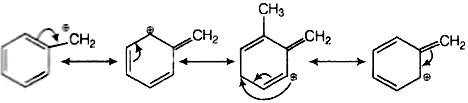Q.24. Which of the carbon atoms present in the molecule given below are asymmetric?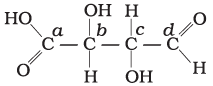(i) a, b, c, d
(ii) b, c
(iii) a, d
(iv) a, b, c
Ans. (ii)
Solution.

Carbon has four valencies. If a carbon atom satisfies all of its four valencies with four different groups then it is termed asymmetric/chiral carbon. In the given compound, b and c carbon are bonded to four different groups, so these are asymmetric.

Q.25. Which of the following compounds will give racemic mixture on nucleophilic substitution by OH  ion?
(a)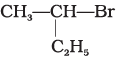(b)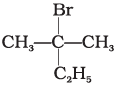(c)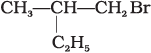(i) (a)
(ii) (a), (b), (c)
(iii) (b), (c)
(iv) (a), (c)
Ans. (i)
Solution.

A mixture containing two enantiomers in equalimolar amount has zero optical rotion as the rotation due to one isomer is cancelled by the rotation due to other isomar. Such mixture is known as racemic mixture. All those compounds which follow SN1 mechanism during nucleophilic substitution reaction form racemic mixture. Order of reactivity halides towards SN1 reactions is as follows:
Tertiary halide > secondary halides > primary halide
Here compound (a) contains a chiral carbon and gives a racemic product. So, it will give racemic mixture.
Hence, option (i) is correct.

Q.26. In the question arrange the compounds in increasing order of rate of reaction towards nucleophilic substitution.
(a)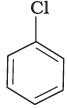(b)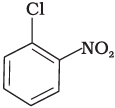(c)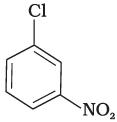(i) (a) < (b) < (c)
(ii) (c) < (b) < (a)
(iii) (a) < (c) < (b)
(iv) (c) < (a) < (b)
Ans. (iii)
Solution.

The bond formed between C of benzene ring and halogen is more stable because of resonance it has partial double bond character. So, rate of reaction towards nucleophilic substitution is slow. This substitution is facilitated by the presence of electron withdrawing group at ortho and para position because electron density is high at these positions.
Compound (b) and (c) both has one electron withdrawing group but in compound (b) electron withdrawing (—NO2) group is present at ortho position, so rate of reaction in compound (b) is more than that of (c) while (a) has no electron withdrawing group.
Hence, the correct option is (iii).

Q.27. In the question arrange the compounds in increasing order of rate of reaction towards nucleophilic substitution.
(a)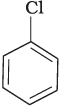(b)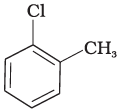(c)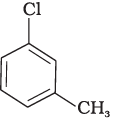(i) (a) < (b) < (c)
(ii) (a) < (c) < (b)
(iii) (c) < (b) < (a)
(iv) (b) < (c) < (a)

Ans. (iv)
Solution.

Presence of electron releasing group ortho or para position decreases the rate of nucleophilic substitution reaction, in compound (c), electron releasing group is present at meta position w.r.t. chlorine BC re impact is less but in compound (b) it is present at ortho position.
Thus, the rate of reaction towards nucleophilic substation is least in compound (b) and highest in compound (a) as there is no electron releasing group in this compound.

Q.28. In the question arrange the compounds in increasing order of rate of reaction towards nucleophilic substitution.
(a)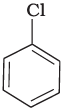(b)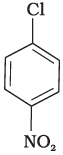(c)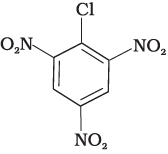(i) (c) < (b) < (a)
(ii) (b) < (c) < (a)
(iii) (a) < (c) < (b)
(iv) (a) < (b) < (c)
Ans. (iv)
Solution.

Presence of electron withdrawing group at ortho and para position facilitate the nucleophilic substitution reaction and hence, enhances rate of reaction.
Compound (c) has three electron withdrawing groups at ortho and para positions w.r.t. chlorine while compound (b) has only one electron withdrawing group and there is no electron withdrawing group in compound (a). So, the increasing order of rate of reaction towards nucleophilic substitution is (a) < (b) < (c).

Q.29. In the question arrange the compounds in increasing order of rate of reaction towards nucleophilic substitution.
(a)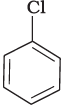(b)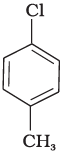(c)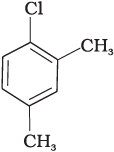(i) (a) < (b) < (c)
(ii) (b) < (a) < (c)
(iii) (c) < (b) < (a)
(iv) (a) < (c) < (b)

Ans. (iii)
Solution.

Presence of electron releasing group at ortho and para position w.r.t. to chlorine decreases the rate of nucleophilic substitution reaction. Compound (c) has two electron releasing groups and compound (b) has one electron releasing group w.r.t. chlorine while compound (a) has no electron releasing group.
So, the rate of nucleophilic substitution reaction is highest in compound (a) and order is (c) < (b) < (a)

Q.30. Which is the correct increasing order of boiling points of the following compounds?
1-Iodobutane, 1-Bromobutane, 1-Chlorobutane, Butane
(i) Butane < 1-Chlorobutane < 1-Bromobutane < 1-Iodobutane
(ii) 1-Iodobutane < 1-Bromobutane < 1-Chlorobutane < Butane
(iii) Butane < 1-Iodobutane < 1-Bromobutane < 1-Chlorobutane
(iv) Butane < 1-Chlorobutane < 1-Iodobutane < 1-Bromobutane

Ans. (i)
Solution.

Higher the surface area, higher will be the intermolecular forces of attraction and thus boiling point too. Boiling point increases with increase in molecular mass of halogen atom for the similar type of alkyl halide. Butane has no halogen atom and rests of all three compounds are halo derivatives of butane.
Atomic mass of iodine is highest so the boiling point of 1-iodobutane is maximum among all the given compounds and hence, option (i) incorrect.

Q.31. Which is the correct increasing order of boiling points of the following compounds?
1-Bromoethane,   1-Bromopropane, 1-Bromobutane, Bromobenzene
(i) Bromobenzene < 1-Bromobutane < 1-Bromopropane < 1-Bromoethane
(ii) Bromobenzene < 1-Bromoethane < 1-Bromopropane < 1-Bromobutane
(iii) 1-Bromopropane < 1-Bromobutane < 1-Bromoethane < Bromobenzene
(iv) 1-Bromoethane < 1-Bromopropane < 1-Bromobutane < Bromobenzene
Ans. (iv)
Solution.

Boiling point increases with increase in size of hydrocarbon part for the same haloalkanes. All the given haloalkanes contain same halogen atom i.e., bromine but the number of carbon atoms in hydrocarbon part of the molecule are increasing from ethane to benzene.
So the boiling point is minimum for 1-bromoethane and maximum for 1-bromobenzene.

MULTIPLE CHOICE QUESTIONS (TYPE - II)

Note : In the following questions two or more options may be correct.
Consider the following reaction and answer the questions no. 32–34.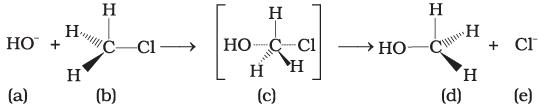Q.32. Which of the statements are correct about above reaction?
(i) (a) and (e) both are nucleophiles.
(ii) In (c) carbon atom is sp3 hybridised.
(iii) In (c) carbon atom is sp2 hybridised.
(iv) (a) and (e) both are electrophiles.

Ans. (i, iii)
Solution.

In the above reaction, OH and Cl both are electron rich species as they are holding the negative charge. So, they are nucleophiles.
The above reaction shows SN2 mechanism, carbon of alkyl halide is sp3 hybridised.
During this mechanism, the breaking of C—X bond and formation of new bond (C—Nu) occurs simultaneously through a transition state in which carbon atom is approximately sp2 hybridised.

(i) The given reaction follows SN2 mechanism.
(ii) (b) and (d) have opposite configuration.
(iii) (b) and (d) have same configuration.
(iv) The given reaction follows SN1 mechanism.
Ans. (i, ii)
Solution.

In the given reaction, alkyl halide is primary in nature Here, a transitory state is observed in which one bond is broken and one bond is formed synchronously i.e., in one step. So, it follows SN2 mechanism. In this mechanism, nucleophile attacks the carbon at 180°: to the leaving group, so the reactant and product have opposite configuration

Q.34. Which of the following statements are correct about the reaction intermediate?
(i) Intermediate (c) is unstable because in this carbon is attached to 5 atoms.
(ii) Intermediate (c) is unstable because carbon atom is sp2 hybridised.
(iii) Intermediate (c) is stable because carbon atom is sp2 hybridised.
(iv) Intermediate (c) is less stable than the reactant (b).

Ans. (i, iv)
Solution.

For the given reaction, intermediate (c) represent transition state, and it is highly unstable. In this transition state, carbon atom is sp2 hybridised as partially bonded to two nucleophiles so it is highly unstable and less stable than the reactant (b) Reactant (b).carbon atom is sp3 hybridised and more stable than intermediate (c).

Answer Q. No. 35 and 36 on the basis of the following reaction.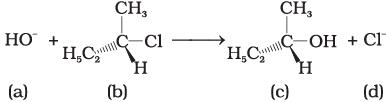Q.35. Which of the following statements are correct about the mechanism of this reaction?
(i) A carbocation will be formed as an intermediate in the reaction.
(ii) OH will attach the substrate (b) from one side and Cl will leave it simultaneously from other side.
(iii) An unstable intermediate will be formed in which OH and Cl will be attached by weak bonds.
(iv) Reaction proceeds through SN1 mechanism.

Ans. (i, iv)
Solution.

The reactant Involve above reaction is secondary alkyl halide. This 2° alkyl halide contain bulky group that’s why it follow SN1 mechanism instead of SN2 mechanism. In SN2 mechanism SN1 mechanism, a stable carbocation will be formed as an intermediate. It is further attacked by OH nucleophile.

Offer running on EduRev: Apply code STAYHOME200 to get INR 200 off on our premium plan EduRev Infinity!

,

,

,

,

,

,

,

,

,

,

,

,

,

,

,

,

,

,

,

,

,

;